### Filter by type: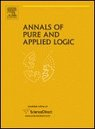#### Computability in Europe 2009

special issues
Klaus Ambos-Spies, Arnold Beckmann, Sam Buss, Benedikt Löwe
special issue of the journal Annals of Pure and Applied Logic, Volume 163, Issue 5
Publication year: 2012#### Computability in Europe 2009

special issues
Arnold Beckmann, Wolfgang Merkle, Benedikt Löwe
special issue of the journal Theory of Computing Systems, Volume 51, Number 1
Publication year: 2012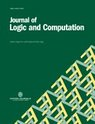#### Computability in Europe 2008

special issues
Arnold Beckmann, Benedikt Löwe
special issue of the journal Journal of Logic and Computation, Volume 22, Number 2
Publication year: 2012

journal
Arnold Beckmann and Sam Buss
TCS 2011, 412(39): 5433-5445
Publication year: 2011

The paper corrects earlier upper bounds on the size of free-cut elimination. Free-cut elimination allows cut elimination to be carried out in the presence of non-logical axioms. The correction requires that the notion of a free-cut be modified so that a cut formula is anchored provided that all of its introductions are anchored, instead of only requiring that one of its introductions is anchored. With the correction, the originally proved size upper bounds remain unchanged. These results also apply to partial cut elimination. We also apply these bounds to elimination of cuts in propositional logic.
If the non-logical inferences are closed under cut and infer only atomic formulas, then all cuts can be eliminated. This generalizes earlier results of Takeuti and of Negri and van Plato.#### Computability in Europe 2008

special issues
Arnold Beckmann, Benedikt Löwe
special issue of the journal Theory of Computing Systems, Volume 48, Number 3
Publication year: 2011

#### On the computational complexity of cut-reduction

journal
Klaus T Aehlig and Arnold Beckmann
APAL 2010, 161(6): 711-736
Publication year: 2010

Using appropriate notation systems for proofs, cut-reduction can often be rendered feasible on these notations, and explicit bounds can be given. Developing a suitable notation system for Bounded Arithmetic, and applying these bounds, all the known results on definable functions of certain such theories can be reobtained in a uniform way.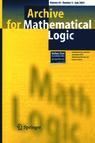#### Computability in Europe 2008

special issues
Arnold Beckmann, Costas Dimitracopoulos, Benedikt Löwe
special issue of the journal Archive for Mathematical Logic, Volume 49, Number 2
Publication year: 2010

#### Characterising Definable Search Problems in Bounded Arithmetic via Proof Notations

journal
Arnold Beckmann and Sam Buss
In: Ways of Proof Theory, ed. R. Schindler, Ontos Series on Mathematical Logic, pp. 65-134, 2010.
Publication year: 2010

The complexity class of Π^p_k – Polynomial Local Search (PLS) problems with Π^p_l – goal is introduced, and is used to give new characterisations of definable search problems in fragments of Bounded Arithmetic. The characterisations are established via notations for propositional proofs obtained by translating Bounded Arithmetic proofs using the Paris-Wilkie-translation. For l ≤ i ≤ k , the Σ bi – definable search problems of T^k+1_2 are exactly characterised by Π^p_k – PLS problems with Π^p_l – goals. These Π^p_k – PLS problems can be defined in a weak base theory such as S^1_2 , and proved to be total in T^k+1_2 . Furthermore, the Π^p_k – PLS definitions can be Skolemised with simple polynomial time functions. The Skolemised Π^p_k – PLS definitions give rise to a new ∀Σ^b_1(α) – principle conjectured to separate T^k_2(α) and T^k+1_2(α).

#### Polynomial Local Search in the Polynomial Hierarchy and Witnessing in Fragments of Bounded Arithmetic

journal
Arnold Beckmann and Sam Buss
Journal of Mathematical Logic 2009, 9(1): 103-138
Publication year: 2009

The complexity class of Π^p_k – polynomial local search (PLS) problems is introduced and is used to give new witnessing theorems for fragments of bounded arithmetic. For 1 ≤ i ≤ k+1 , the Σ^b_i – definable functions of T^k+1_2 are characterized in terms of Π^p_k – PLS problems. These Π^p_k – PLS problems can be defined in a weak base theory such as S^1_2 , and proved to be total in T^k+1_2 . Furthermore, the Π^p_k – PLS definitions can be skolemized with simple polynomial time functions, and the witnessing theorem itself can be formalized, and skolemized, in a weak base theory. We introduce a new ∀Σ^b_1(α) – principle that is conjectured to separate T^k_2(α) and T^k+1_2(α) .

#### A Characterisation of Definable NP Search Problems in Peano Arithmetic

conference
Arnold Beckmann
Logic, Language, Information and Computation. 16th International Workshop, WoLLIC 2009, Tokyo, Japan, June 21-24, 2009. Pages: 1 - 12
Publication year: 2009

The complexity class of \$\prec\$-bounded local search problems with goals is introduced for well-orderings \$\prec\$, and is used to give a characterisation of definable NP search problems in Peano Arithmetic.

#### On the computational complexity of cut-reduction

conference
Klaus T Aehlig and Arnold Beckmann
Logic in Computer Science, 2008. LICS '08. 23rd Annual IEEE Symposium on 24-27 June 2008. Pages: 284 - 293
Publication year: 2008

Using appropriate notation systems for proofs, cut-reduction can often be rendered feasible on these notations, and explicit bounds can be given. Developing a suitable notation system for Bounded Arithmetic, and applying these bounds, all the known results on definable functions of certain such theories can be reobtained in a uniform way.

#### On the complexity of parity games

conference
Arnold Beckmann and Faron G Moller
Visions of Computer Science — BCS International Academic Conference Imperial College, London, UK - 22 - 24 September 2008. Pages: 237 - 247
Publication year: 2008

Parity games underlie the model checking problem for the modal μ-calculus, the complexity of which remains unresolved after more than two decades of intensive research. The community is split into those who believe this problem – which is known to be both in NP and coNP – has a polynomial-time solution (without the assumption that P=NP) and those who believe that it does not. (A third, pessimistic, faction believes that the answer to this question will remain unknown in their lifetime.)

In this paper we explore the possibility of employing Bounded Arithmetic to resolve this question, motivated by the fact that problems which are both NP and coNP, and where the equivalence between their NP and coNP description can be formulated and proved within a certain fragment of Bounded Arithmetic, necessarily admit a polynomial-time solution. While the problem remains unresolved by this paper, we do proposed another approach, and at the very least provide a modest refinement to the complexity of parity games (and in turn the μ-calculus model checking problem): that they lie in the class of Polynomial Local Search problems. This result is based on a new proof of memoryless determinacy which can be formalised in Bounded Arithmetic.

The approach we propose may offer a route to a polynomial-time solution. Alternatively, there may be scope in devising a reduction between the problem and some other problem which is hard with respect to PLS, thus making the discovery of a polynomial-time solution unlikely according to current wisdom.#### Logic and Theory of Algorithms

edited volumes
Arnold Beckmann, Costas Dimitracopoulos, Benedikt Löwe
Fourth Conference on Computability in Europe, CiE 2008, Athens, Greece, June 2008, Proceedings, LNCS 5028
Publication year: 2008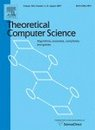#### From Gödel to Einstein: Computability between Logic and Physics at CiE 2006

special issues
Arnold Beckmann, Edwin Beggs, Benedikt Löwe
special issue of the journal Theoretical Computer Science A, Volume 394, Issue 3
Publication year: 2008

#### Continuous Fraïssé Conjecture

journal
Arnold Beckmann, Martin Goldstern and Norbert Preining
Order 2008, 25: 281-298
Publication year: 2008

We investigate the relation of countable closed linear orderings with respect to continuous monotone embeddability and show that there are exactly ℵ1 many equivalence classes with respect to this embeddability relation. This is an extension of Laver’s result, who considered (plain) embeddability, which yields coarser equivalence classes. Using this result we show that there are only ℵ0 many different Gödel logics.#### Computability in Europe 2006

special issues
Arnold Beckmann, Benedikt Löwe
special issue of the journal Theory of Computing Systems, Volume 43, Issue 3-4
Publication year: 2008

#### Propositional Logic for Circuit Classes

conference
Klaus T Aehlig and Arnold Beckmann
21st International Workshop, CSL 2007, 16th Annual Conference of the EACSL, Lausanne, Switzerland, September 11-15, 2007. Pages: 512 - 526
Publication year: 2007

By introducing a parallel extension rule that is aware of independence of the introduced extension variables, a calculus for quantified propositional logic is obtained where heights of derivations correspond to heights of appropriate circuits. Adding an uninterpreted predicate on bit-strings (analog to an oracle in relativised complexity classes) this statement can be made precise in the sense that the height of the most shallow proof that a circuit can be evaluated is, up to an additive constant, the height of that circuit.

The main tool for showing lower bounds on proof heights is a variant of an iteration principle studied by Takeuti. This reformulation might be of independent interest, as it allows for polynomial size formulae in the relativised language that require proofs of exponential height.#### Logical Approaches to Computational Barriers: CiE 2006

special issues
Arnold Beckmann, Benedikt Löwe, Dag Normann
special issue of the journal Journal of Logic and Computation, Volume 17, Issue 6.
Publication year: 2007

#### Linear Kripke Frames and Gödel Logics

journal
Arnold Beckmann and Norbert Preining
The Journal of Symbolic Logic Vol. 72, No. 1, pp. 26-44
Publication year: 2007

We investigate the relation between logics of countable linear Kripke frames with constant domains and Gödel logics. We show that for any such Kripke frame there is a Gödel logic which coincides with the logic of this Kripke frame and vice versa. This allows us to transfer several recent results on Gödel logics to the logics of countable linear Kripke frames with constant domains.#### Logical Approaches to Computational Barriers

edited volumes
Arnold Beckmann, Ulrich Berger, Benedikt Löwe, John V. Tucker
Second Conference on Computability in Europe, CiE 2006, Swansea, UK, July 2006, Proceedings, LNCS 3988
Publication year: 2006

#### Generalised dynamic ordinals - universal measures for implicit computational complexity

journal
Arnold Beckmann
Logic Colloquium '02, Lecture Notes in Logic 27: 48-74, Association for Symbolic Logic, La Jolla, CA, 2006.
Publication year: 2006

We extend the definition of dynamic ordinals to generalised dynamic ordinals. We compute generalised dynamic ordinals of all fragments of relativised bounded arithmetic by utilising methods from Boolean complexity theory, similar to [Krajicek1994]. We indicate the role of generalised dynamic ordinals as universal measures for implicit computational complexity. I.e., we describe the connections between generalised dynamic ordinals and witness oracle Turing machines for bounded arithmetic theories. In particular, through the determination of generalised dynamic ordinals we re-obtain well-known independence results for relativised bounded arithmetic theories.

#### Uniform proof complexity

journal
Arnold Beckmann
Journal of Logic and Computation 2005, 15: 433-446
Publication year: 2005

We define the notion of the uniform reduct of a propositional proof system as the set of those bounded formulas in the language of Peano Arithmetic which have polynomial size proofs under the Paris-Wilkie-translation. With respect to the arithmetic complexity of uniform reducts, we show that uniform reducts are Π^0_1-hard and obviously in Σ^0_2. We also show under certain regularity conditions that each uniform reduct is closed under bounded generalisation; that in the case the language includes a symbol for exponentiation, a uniform reduct is closed under modus ponens if and only if it already contains all true bounded formulas; and that each uniform reduct contains all true Π^b_1(α)-formulas.

#### Separation results for the size of constant-depth propositional proofs

journal
Arnold Beckmann and Sam Buss
Annals of Pure and Applied Logic 2005, 136: 30-55
Publication year: 2005

This paper proves exponential separations between depth d-LK and depth (d+1/2)-LK for every d in 0, 1/2, 1, 1 1/2,… utilizing the order induction principle. As a consequence, we obtain an exponential separation between depth d-LK and depth (d+1)-LK for d in 0,1,2,… . We investigate the relationship between the sequence-size, tree-size and height of depth d-LK-derivations for d in 0, 1/2, 1, 1 1/2,… and describe transformations between them.

We define a general method to lift principles requiring exponential tree-size (d+1/2)-LK-refutations for d in 0,1,2,… to principles requiring exponential sequence-size d-LK-refutations, which will be described for the Ramsey principle and d=0. From this we also deduce width lower bounds for resolution refutations of the Ramsey principle.#### Festschrift on the occasion of Wolfram Pohlers 60th birthday

special issues
Arnold Beckmann, Jeremy Avigad, Georg Moser
special issue of the journal Annals of Pure and Applied Logic, Volume 136, Issues 1-2, Pages 1-218
Publication year: 2005

#### An Unexpected Separation Result in Linearly Bounded Arithmetic

journal
Arnold Beckmann and Jan Johannsen
Math. Log. Quart. 2005, 51: 191-200
Publication year: 2005

The theories S^i_1(α) and T^i_1(α) are the analogues of Buss’ relativized bounded arithmetic theories in the language where every term is bounded by a polynomial, and thus all definable functions grow linearly in length. For every i , a Σ^b_i+1(α) – formula TOP^i(α), which expresses a form of the total ordering principle, is exhibited that is provable in S^i+1_1(α) , but unprovable in T^i_1(α). This is in contrast with the classical situation, where S^i+1_2 is conservative over T^i_2 w.r.t. Σ^b_i+1-sentences. The independence results are proved by translations into propositional logic, and using lower bounds for corresponding propositional proof systems.

#### Preservation theorems and restricted consistency statements in bounded arithmetic

journal
Arnold Beckmann
Ann.Pure.Appl.Logic 2004, 126: 255-280
Publication year: 2004

We define and study a new restricted consistency notion for bounded arithmetic theories T2j. It is the strongest ∀Π1b-statement over S21 provable in T2j, similar to Con(Gi) in Krajíček and Pudlák, (Z. Math. Logik Grundl. Math. 36 (1990) 29) or RCon(Ti1) in Krajı́ček and Takeuti (Ann. Math. Artificial Intelligence 6 (1992) 107). The advantage of our notion over the others is that can directly be used to construct models of T2j. We apply this by proving preservation theorems for theories of bounded arithmetic of the following well-known kind: The ∀Π1b-separation of bounded arithmetic theories S2i from T2j (1⩽i⩽j) is equivalent to the existence of a model of S2i which does not have a Δ0b-elementary extension to a model of T2j.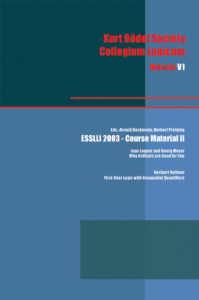#### ESSLLI 2003 - Course Material II

edited volumes
Arnold Beckmann, Norbert Preining
Collegium Logicum Volume 6, KGS Wien, 2004, VIII+105pp, ISBN 3-901546-01-4.
Publication year: 2004

Ingo Lepper and Georg Moser: Why Ordinals are Good for You;
Heribert Vollmer: First-Order Logic with Groupoidal Quantifiers.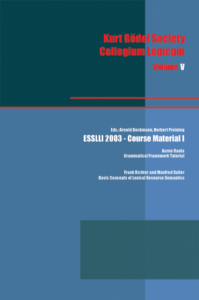#### ESSLLI 2003 - Course Material I

edited volumes
Arnold Beckmann, Norbert Preining
Collegium Logicum Volume 5: ESSLLI 2003 - Course Material I; KGS Wien, 2004, VIII+143pp, ISBN 3-901546-00-6.
Publication year: 2004

Aarne Ranta: Grammatical Framework Tutorial;
Frank Richter and Manfred Sailer: Basic Concepts of Lexical Resource Semantics.

#### Bounded Arithmetic and Resolution-Based Proof Systems

book
Arnold Beckmann and Jan Johannsen